In :
from IPython.display import HTML

HTML('''<script>
code_show=true;
function code_toggle() {
if (code_show){
$('div.input').hide(); } else {$('div.input').show();
}
code_show = !code_show
}
</script>

Out:

In many machine learning problems your dataset is simply a collection of observations or features. The outcome for a new datapoint is predicted simply based on the value of its features: the order of the data is not important.

Time series data is different.

Time series adds an order dependence between observations. This additional dimension is both a constraint and a structure that provides a source of additional information. Using time series analysis tools we can describe data - in terms of seasonal patterns, trends, relation to external factors or events etc. - and predict the future.

One common example of a time series model is the autoregressive integrated moving average (ARIMA) model. There are a host of open source tools available now for doing time series analysis, including Facebook's recently open-sourced Prophet. Prophet uses an additive regression model and is designed to be usable with minimal effort: perfect for us to have some fun with!

So, now we need some data! The crime datasets made available at data.police.uk under the open government licence are perfect for this. The dataset includes crime and outcomes data from all the police forces in England and Wales, the British Transport Police and the Police Service of Northern Ireland.

The crime dataset includes some granular location data (longitude, latitude, street), but for this post we're going to keep it simple and just look at crimes reported by month, area and type since September 2015 (a dataset of just under 20 million incidents).

In :
import os, datetime
import pandas as pd
import numpy as np
import matplotlib
import matplotlib.pyplot as plt
from statsmodels.graphics.tsaplots import plot_acf
from fbprophet import Prophet
from pandas.plotting import _converter
_converter.register()
import warnings
warnings.filterwarnings('ignore')

In :
# load in raw data from police files (stored in the *data* directory)
data = pd.DataFrame()
for month in os.listdir("data"):
for file in os.listdir("data/" + month):
data = pd.concat([data,pd.read_csv("data/" + month + "/" + file, usecols=['Month','Reported by','Crime type'])])

# parse date field, and drop incomplete months
data['Month'] = pd.to_datetime(data['Month'])
data = data[(data['Month'] > pd.Timestamp(datetime.date(2015,9,1))) & (data['Month'] < pd.Timestamp(datetime.date(2018,8,1)))]

In :
# let's have a look at roughly what the data looks like
data.sample(n=10)

Out:
Month Reported by Crime type
6698 2015-10-01 Sussex Police Anti-social behaviour
12957 2015-10-01 Merseyside Police Other theft
1690 2018-03-01 South Wales Police Anti-social behaviour
20358 2016-01-01 West Yorkshire Police Violence and sexual offences
2851 2016-04-01 Warwickshire Police Criminal damage and arson
11636 2016-02-01 Metropolitan Police Service Theft from the person
26573 2017-01-01 Metropolitan Police Service Theft from the person
11283 2015-12-01 South Yorkshire Police Criminal damage and arson
65485 2018-07-01 Metropolitan Police Service Violence and sexual offences
3013 2017-07-01 Leicestershire Police Anti-social behaviour

## Autocorrelation¶

Before we dive into using Prophet, a good statistical measure of the internal correlation within a time series is autocorrelation. Autocorrelation is the correlation of a signal with a delayed copy of itself as a function of delay. For non time series data there should be no pattern in the autocorrelation as the order of observations is not relevent, however for time series data we should see some correlations.

In :
fig,ax = plt.subplots()

# bucket data by date (granularity is months)
dataByMonth = data.groupby(['Month']).size().reset_index(name='counts')
plot_acf(dataByMonth['counts'], lags=30, ax=ax);
plt.show();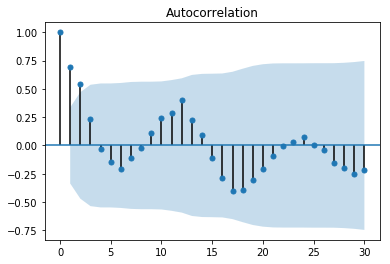For our crime data adjacent observations are clearly correlated, and we can also see some seasonality with what looks like a periodicity of 12 i.e. yearly seasonality.

## Use Prophet to predict the future!¶

As mentioned before, one of the main uses of modelling on time series data is predicting the future. Prophet makes it fairly straightforward to find seasonality and underlying trends in your data, and predict future dates based on these patterns. So let's try predicting the next 12 months.

In :
fig,ax = plt.subplots(figsize=(10,6))

# Prophet expects certain column names
dataByMonth.columns = ['ds','y']

# and fit a simple prophet model, and predict 12 moths into the future
m = Prophet(weekly_seasonality=False, daily_seasonality=False, seasonality_mode='multiplicative', seasonality_prior_scale=0.05).fit(dataByMonth)
forecast = m.predict(m.make_future_dataframe(periods=12,freq='M'))
m.plot(forecast, ax=ax)
ax.axvspan(dataByMonth['ds'].max(), forecast['ds'].max(), alpha=0.15, color='black')
ax.set_ylabel('Number of crimes')
ax.set_xlabel('Month')
plt.show();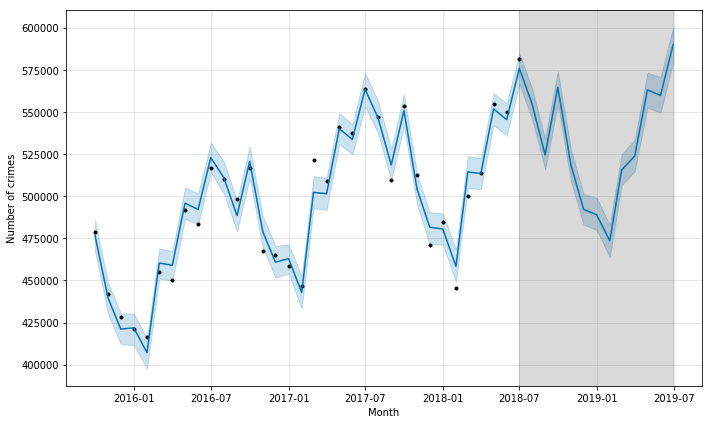Here the black points are actual data, the blue line is the prediction, the blue shaded area is the uncertainty in the prediction and the gray shaded area is the future. There is clearly an underlying trend, and some major seasonal patterns in the data, which prophet extrapolates into the future.

## Let's dig into the details...¶

So we can try to predict the future with these tools. However, it can also be very interesting and valuable to dig into the patterns the model finds i.e. how is it describing the data?

Time series models include seasonal componenents over a range of horizons such as days, weeks, or years; for example your data might predictably spike on Saturdays. As well as these seasonal components the model will identify underlying trends. In the prediction above we can clealy see yearly seasonality in our data - with more crime in the summer - and an overall upwards trend.

To look at this in more detail let's split the crime data up and model each type of crime separately, then look at the yearly seasonal (on the left) and underlying trend components (on the right) of our model. The seasonal plots show how much crime increases or decreases from average in each month, and the underlying trend shows how counts of crime have changed relative to 2015 (and how they are predicted to continue to change in future, shaded in grey).

In :
# extract list of types, and setup empty dataframes
types = data['Crime type'].unique()
trendByType = pd.DataFrame()
seasonaltrendByType = pd.DataFrame()

# gets counts of incidents by month and type
dataByMonthAndType = data.groupby(['Month','Crime type']).size().reset_index(name='counts')

for typ in types:
# pull out data for only this type, and build a model
d = dataByMonthAndType[dataByMonthAndType['Crime type'] == typ][['Month','counts']]
d.columns = ['ds','y']
m = Prophet(weekly_seasonality=False, daily_seasonality=False).fit(d)

# predict 18 months into future and extract seasonal trend
trend = m.make_future_dataframe(periods=18,freq='M')
trend['y'] = m.predict(trend)['trend']
trend['Type'] = typ
trendByType = pd.concat([trendByType,trend])
seasonalTrend = pd.DataFrame({'month': pd.date_range(start='2017-01-01', periods=12, freq='MS')})
seasonalTrend['y'] = m.predict_seasonal_components(m.seasonality_plot_df(seasonalTrend['month']))['yearly']
seasonalTrend['Type'] = typ
seasonaltrendByType = pd.concat([seasonaltrendByType,seasonalTrend])

In :
# setup plot
fig,ax = plt.subplots(ncols=2, nrows=len(types), figsize=(12,4*len(types)))

# plot the seasonal trend and yearly trend by crime type
for row,typ in zip(ax,types):
# extract the seasonality as % dev from average, and trend as % dev from start
seasonalTrend = seasonaltrendByType[seasonaltrendByType['Type'] == typ]
trend = trendByType[trendByType['Type'] == typ]
seasonalTrend['y'] = seasonalTrend['y'] / trend['y'].mean()
trend['y'] = trend['y'] / trend['y']

row.scatter(seasonalTrend['month'].values, seasonalTrend['y'].values, s=100, alpha=0.5)
row.set_xlim([np.datetime64('2016-12-01'),np.datetime64('2018-01-01')])
row.set_ylim([-0.5,0.5])
row.xaxis.set_major_formatter(matplotlib.dates.DateFormatter('%b'))
row.yaxis.set_major_formatter(matplotlib.ticker.FuncFormatter(lambda y, _: '{:.0%}'.format(y)))
row.set_ylabel('seasonal change')
row.plot(trend['ds'],-1+trend['y'], linestyle='None', marker='.',markersize=5)
row.set_ylim([-1,2])
row.yaxis.set_major_formatter(matplotlib.ticker.FuncFormatter(lambda y, _: '{:.0%}'.format(y)))
row.axvspan(dataByMonth['ds'].max(), trend['ds'].max(), alpha=0.15, color='black')
row.set_title(typ)
row.text(dataByMonth['ds'].min(), 1.7, "{} is {:.0%} of total crime".format(typ,trendByType[trendByType['Type'] == typ]['y'].sum() / trendByType['y'].sum()))
row.set_ylabel('underlying change (from 2015)')
row.tick_params(axis='x', rotation=30)

plt.show();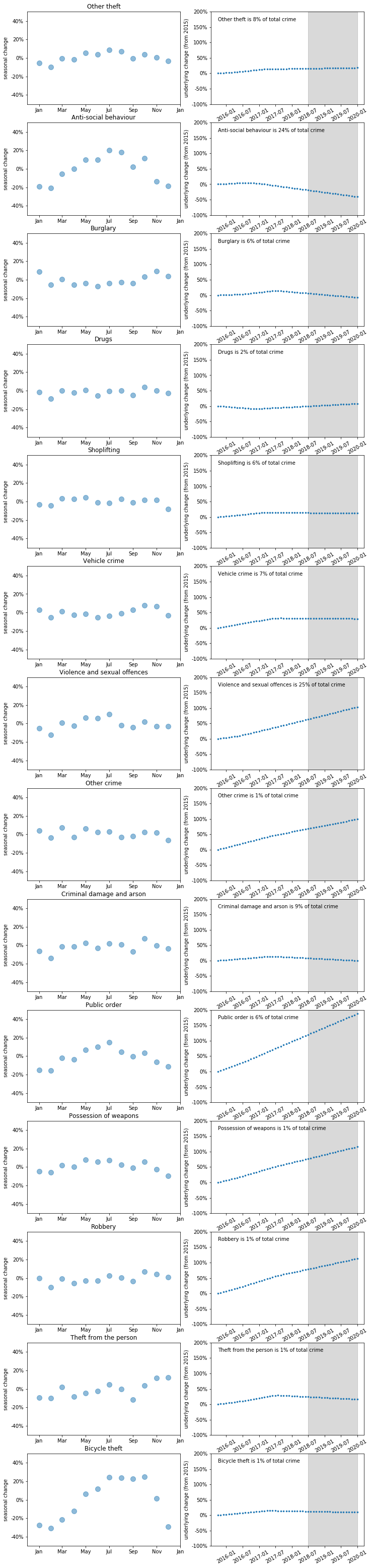So this is pretty interesting! Some crime types are clearly much more seasonal than others, and overall trends vary alot:

• Anti-social behaviour and bicycle theft are much more common in the summer months, which makes sense since these are crimes are generally commited outside
• Burglary is slightly more common in the winter months
• Anti-social behaviour has fallen alot in the last few years, while violent crime is up (this may be due to reporting differences)

To get a better picture of the overall makeup of reported crime we can look at the same data in stacked area plots, which makes it clear violent crime and anti-social beavhiour are the two dominant types, and have contrasting upwards and downwards trends almost balancing each other.

In :
fig,ax = plt.subplots(ncols=2, figsize=(16,6))

ax.stackplot(seasonaltrendByType['month'].unique(), *[trendByType[trendByType['Type'] == typ]['y'].mean() + seasonaltrendByType[seasonaltrendByType['Type'] == typ]['y'] for typ in types], labels = types)
handles, labels = ax.get_legend_handles_labels()
ax.legend(reversed(handles), reversed(labels), loc='best', framealpha=0.5)
ax.xaxis.set_major_formatter(matplotlib.dates.DateFormatter('%b'))
ax.set_title('Seasonal trend')
ax.stackplot(trendByType['ds'].unique(), *[trendByType[trendByType['Type'] == typ]['y'] for typ in trendByType['Type'].unique()], labels = trendByType['Type'].unique())
ax.set_title('Overall trend')
ax.axvspan(dataByMonth['ds'].max(), trendByType['ds'].max(), alpha=0.15, color='black')

fig.autofmt_xdate()
plt.show();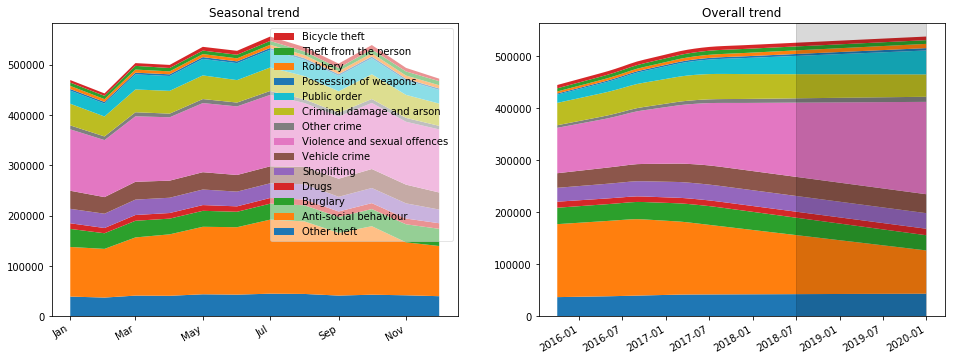## We can dig even deeper¶

So as well as spliting the data up by type, we can also look at the differences in crime across the different areas in our dataset. To make this data easier to visualize and interpret the crime in each area is reduced to two simple metrics:

• How much of this crime is there compared to the national average? Where 100% is the average
• The seasonality: how much more crime is there in the summer than the winter months

To more clearly illustrate what we mean by seasonality:

In :
fig,ax = plt.subplots(figsize=(10,6))

seasonalTrend = seasonaltrendByType[seasonaltrendByType['Type'] == 'Bicycle theft']
bike_thefts = seasonalTrend['y'].values + trendByType[trendByType['Type'] == 'Bicycle theft']['y'].mean()
ax.scatter(seasonalTrend['month'].values, bike_thefts, s=100, alpha=0.5)
ax.scatter(seasonalTrend['month'].values[:2], bike_thefts[:2], s=100, alpha=0.5, color='red')
ax.scatter(seasonalTrend['month'].values[6:8], bike_thefts[6:8], s=100, alpha=0.5, color='green')
ax.set_xlim([np.datetime64('2016-12-01'),np.datetime64('2018-01-01')])
ax.xaxis.set_major_formatter(matplotlib.dates.DateFormatter('%b'))
ax.set_ylabel('Average number of biycle thefts by month')
ax.text(seasonalTrend['month'].min(), 8000, 'Seasonality is the ratio of the average\nsummer value (in green) to average\nwinter value (in red)\n\nIn this case 175%')

plt.show();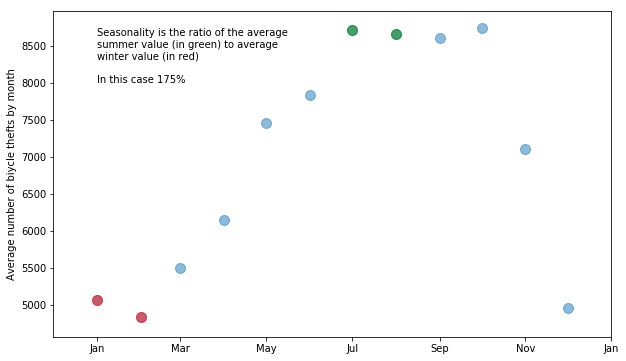In :
dataByMonthAndTypeAndArea = data.groupby(['Month','Crime type','Reported by']).size().reset_index(name='counts')
trendsByMonthAndTypeAndArea = pd.DataFrame()

for typ in types:
for area in dataByMonthAndTypeAndArea['Reported by'].unique():
dataForTypeAndArea = dataByMonthAndTypeAndArea[(dataByMonthAndTypeAndArea['Crime type'] == typ) & (dataByMonthAndTypeAndArea['Reported by'] == area)][['Month','counts']]
dataForTypeAndArea.columns = ['ds','y']
m = Prophet(weekly_seasonality=False, daily_seasonality=False).fit(dataForTypeAndArea)

seasonalTrend = pd.DataFrame({'month': pd.date_range(start='2017-01-01', periods=12, freq='MS')})
trend = m.make_future_dataframe(periods=12,freq='M')
trend['y'] = m.predict(trend)['trend']
seasonalTrend['y'] = m.predict_seasonal_components(m.seasonality_plot_df(seasonalTrend['month']))['yearly']

avg = trend['y'].mean()
seasonality = (seasonalTrend['y'] + avg)[[6,7]].mean() / (seasonalTrend['y'] + avg)[[0,1]].mean()
trendsByMonthAndTypeAndArea = pd.concat([trendsByMonthAndTypeAndArea,pd.DataFrame({'Type':[typ], 'Area':[area], 'avg_count':[avg], 'seasonality':[seasonality]})])

In :
# add some extra stats -> how much does % of a crime type in each area differ from the norm?
trendsByMonthAndTypeAndArea['area_count'] = trendsByMonthAndTypeAndArea[['Area','avg_count']].groupby(['Area']).transform(np.sum)
trendsByMonthAndTypeAndArea['avg_perc'] = trendsByMonthAndTypeAndArea['avg_count'] / trendsByMonthAndTypeAndArea['area_count']
trendsByMonthAndTypeAndArea['type_count'] = trendsByMonthAndTypeAndArea[['Type','avg_count']].groupby(['Type']).transform(np.sum)
trendsByMonthAndTypeAndArea['type_avg_perc'] = trendsByMonthAndTypeAndArea['type_count'] / trendsByMonthAndTypeAndArea['avg_count'].sum()
trendsByMonthAndTypeAndArea['diff_avg'] = trendsByMonthAndTypeAndArea['avg_perc'] / trendsByMonthAndTypeAndArea['type_avg_perc']


So first let's look at how incidence of different crimes varies across the UK; the best tool for this job is a heat map.

In :
# pivot out difference from the average by area
diffFromAvg = trendsByMonthAndTypeAndArea.pivot(index='Area', columns='Type', values='diff_avg')

fig, ax = plt.subplots(figsize=(20,20))
mtrx = diffFromAvg.as_matrix()
im = ax.imshow(mtrx, vmin=0, vmax=3)

# We want to show all ticks...
ax.set_xticks(np.arange(len(diffFromAvg.columns)))
ax.set_yticks(np.arange(len(diffFromAvg.index)))
# ... and label them with the respective list entries
ax.set_xticklabels(diffFromAvg.columns)
ax.set_yticklabels(diffFromAvg.index)

# Rotate the tick labels and set their alignment.
plt.setp(ax.get_xticklabels(), rotation=45, ha="right",
rotation_mode="anchor")

# Loop over data dimensions and create text annotations.
for i in range(len(diffFromAvg.index)):
for j in range(len(diffFromAvg.columns)):
text = ax.text(j, i, '{:.0%}'.format(mtrx[i, j]),
ha="center", va="center", color="w")

ax.set_title("Difference of crime reports to average")
plt.show()